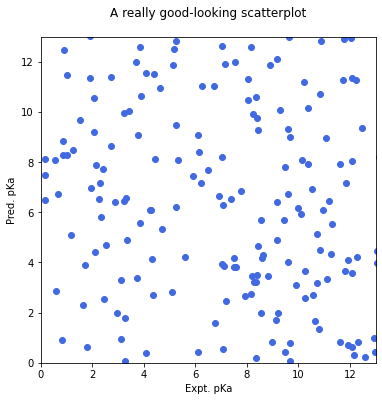# 5 Minutes of Fame¶

#### This week's episode: A small introduction to TikZ and tikzplotlib¶

• TikZ is a LaTeX package that allows the user to draw and generate plots within the source code of your LaTeX document. It can be used for simple things like drawing different shapes, but it can also be used for far more complex tasks
• tikzplotlib is a Python tool with which matplotlib figures can easily and directly be converted into PGFPlots (TikZ), ready to use in your LaTeX document
• This is only one part of the 5 minutes, there is also a corresponding LaTeX project!
In :
```pip install tikzplotlib
```

### Imports¶

In :
```import matplotlib.pyplot as plt
import numpy as np
import tikzplotlib
import random
```

In :
```data1 = np.random.uniform(low=0,high=14,size=(200,))
data2 = np.random.uniform(low=0,high=14,size=(200,))
data3 = random.sample(range(0,1000), 4)
data4 = random.sample(range(0,1000), 4)
```

### Then plot it in a nice fashion and immediately save it as tikzpicture¶

In :
```f1, ax = plt.subplots(figsize=(6,6))
ax.scatter(data1, data2, c='royalblue')
plt.xlabel('Expt. pKa')
plt.ylabel('Pred. pKa')
plt.ylim(0, 13)
plt.xlim(0, 13)

tikzplotlib.save('Scatterplot.tex')                        # This one here is the important line of code!
```### Let's try this one more time, just for fun¶

In :
```# Bar graph for datasets 3 and 4
X = np.arange(4)

labels = ['18-25', '26-39', '40-49', '50-65']
fig1, ax = plt.subplots()
ax.bar(X + 0.00, data3, color = 'orangered', width = 0.25)
ax.bar(X + 0.25, data4, color = 'forestgreen', width = 0.25)
plt.xticks(X + 0.125, labels, rotation=60)
plt.ylabel('Amount of problems')
plt.xlabel('Age')

tikzplotlib.save('Bargraph.tex')
```In [ ]:
```
```